# NR Intercell Interference Modeling

This example shows the impact on network performance due to downlink (DL) intercell interference caused by nearby cells. The example models a 5G New Radio (NR) network of multiple cells operating in the same frequency band. Each cell has a gNB placed at the center of the cell that serves a set of user equipment (UE). The NR stack on the nodes includes radio link control (RLC), medium access control (MAC), and physical layers (PHY).

### Introduction

The example considers these operations at gNB and UEs to facilitate DL transmissions and receptions.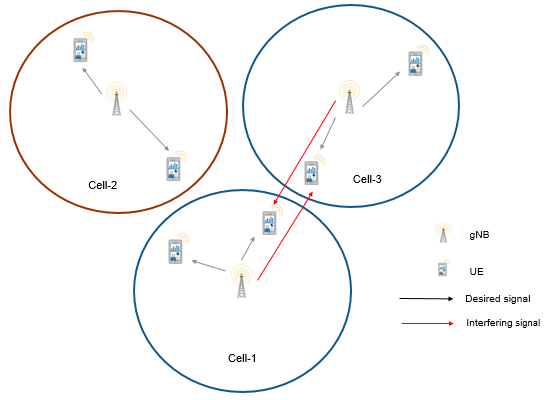The complete PDSCH packet is transmitted in the first symbol of its allocated symbol set. Receiver processes the packet in the symbol just after the last symbol in the allocated symbol set.

This example models:

• Co-channel intercell interference.

• Slot-based and symbol-based DL scheduling of PDSCH resources.

• Configurable subcarrier spacing resulting in different slot durations.

• Noncontiguous allocation of frequency-domain resources in terms of resource block groups (RBGs).

• PDSCH demodulation reference signal (DM-RS).

• DL channel quality measurement by UEs based on the CSI-RS received from gNB. By default, the CSI-RS resource element is transmitted in each slot for each resource block (RB) in DL bandwidth, for all UEs. The same CSI-RS configuration is applicable to all the UEs in the cell.

• Free space path loss (FSPL), and additive white Gaussian noise (AWGN).

• Single input single output (SISO) antenna configuration.

• Single bandwidth part across the whole carrier.

The example assumes that control packets such as DL assignment, PDSCH feedback, and channel quality indicator (CQI) report, are assumed to be sent out of band, that is, without the need of resources for transmission and assured error-free reception.

### Co-Channel Interference

In cellular systems, each cell operates on a particular carrier frequency. The cells operating on the same carrier frequency are called co-channel cells. Co-channel cells can interfere in transmissions between them.

Consider this sample network topology consisting of 3 cells. Cell-1 and cell-3 operate on the same frequency band. Cell-2 operates on a different frequency band and does not interfere with cell-1 or cell-3.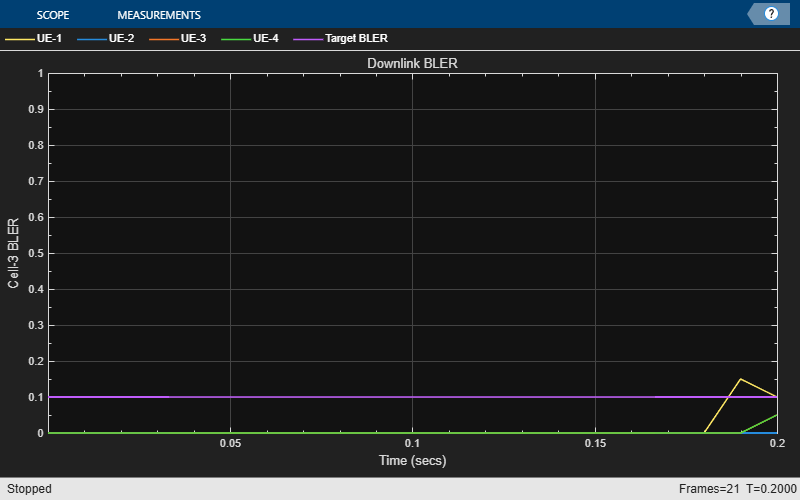#### NR Protocol Stack

A node (gNB or UE) consists of applications generating traffic, in addition to RLC, MAC and PHY. The helper classes hNRGNB.m and hNRUE.m create gNB and UE nodes respectively, containing RLC, MAC, and PHY layers. For more details on each layer, refer to the NR Cell Performance Evaluation with Physical Layer Integration example.

### Scenario Configuration

For this simulation, set the following key configuration parameters.

• Simulation time

• Number of cells

• Cell radius (all the UEs connected to a gNB are within this distance)

• Positions of gNBs

• Number of UEs in each cell

• Signal to interference and noise ratio (SINR) to CQI mapping table for 0.1 block error rate (BLER). The lookup table is used to map the received SINR to CQI index for 0.1 BLER. The lookup table corresponds to the CQI table as per 3GPP TS 38.214 Table 5.2.2.1-3. For more information about the process of generating this lookup table, see the 5G NR Downlink CSI Reporting example.

• Transmit power of gNB

• DL carrier bandwidth in terms of number of resource blocks (RBs)

• DL carrier frequency

• Subcarrier spacing

• DL application traffic model

Configure simulation parameters in the `simParameters` structure.

```rng('default'); % Reset the random number generator simParameters = []; % Clear the simParameters variable simParameters.NumFramesSim = 20; % Simulation time in terms of number of 10 ms frames simParameters.SchedulingType = 0; % Set the value to 0 (slot-based scheduling) or 1 (symbol-based scheduling)```

Specify the number of cells in the simulation. Cells are assumed to have sequential cell identifiers (NCellIDs) from `0` to` NumCells-1`. If you change the number of cells, ensure that the number of rows in `simParameters.GNBPosition` must be equal to `NumCells`.

```simParameters.NumCells = 3; % Number of cells simParameters.CellRadius = 500; % Radius of each cell (in meters) % Validate the number of cells validateattributes(simParameters.NumCells, {'numeric'}, {'nonempty', 'integer', 'scalar', '>', 0, '<=', 1008}, 'simParameters.NumCells', 'NumCells'); % Validate the cell radius validateattributes(simParameters.CellRadius, {'numeric'}, {'nonempty', 'real', 'scalar', '>', 0, 'finite'}, 'simParameters.CellRadius', 'CellRadius');```

The `N-by-3` matrix represents the position of gNBs in (X, Y, Z) coordinates, where '`N`' is the number of cells in the simulation. The row number '`P`' represents the X, Y, and Z coordinates of the gNB in the cell having cell ID '`P-1`'. For example, the value [3000, 600, 0] in the 2nd row represents the (X, Y, Z) coordinates of the gNB in the cell having cell ID 1.

```simParameters.GNBPosition = [1700 600 0; 3000 600 0; 2500 2000 0]; % Validate the gNB positions validateattributes(simParameters.GNBPosition, {'numeric'}, {'nonempty', 'real', 'nrows', simParameters.NumCells, 'ncols', 3, 'finite'}, 'simParameters.GNBPosition', 'GNBPosition');```

Specify the number of UEs in a cell. Each cell contains same number of UEs.

```simParameters.NumUEs = 4; % Validate the number of UEs in each cell validateattributes(simParameters.NumUEs, {'numeric'}, {'nonempty', 'integer', 'scalar','>', 0, '<=', 65519}, 'simParameters.NumUEs', 'NumUEs');```

Specify the gNB transmit power in dBm.

`simParameters.GNBTxPower = 32;`

Set the channel bandwidth to 10 MHz and subcarrier spacing (SCS) to 30 kHz as defined in 3GPP TS 38.104 Section 5.3.2.

```simParameters.NumRBs = 24; simParameters.SCS = 30; % kHz simParameters.DLCarrierFreq = 2.635e9; % DL carrier frequency in Hz simParameters.DLBandwidth = 10e6; % DL bandwidth in Hz ```

Specify the SINR to CQI mapping table for a BLER of 0.1.

```simParameters.SINR90pc = [-5.46 -0.46 4.54 9.05 11.54 14.04 15.54 18.04 ... 20.04 22.43 24.93 25.43 27.43 30.43 33.43];```

Specify the scheduling strategy and the maximum limit on the RBs allotted for PDSCH. The transmission limit applies only to new PDSCH assignments, and not to the retransmissions.

`simParameters.RBAllocationLimitDL = 15;`

#### Logging and visualization configuration

Specify the ID of cell of interest. All the visualizations and metrics are shown for this cell.

```simParameters.CellOfInterest = 2; % Set a value from 0 to NumCells-1 % Validate Cell of interest validateattributes(simParameters.CellOfInterest, {'numeric'}, {'nonempty', 'integer', 'scalar', '>=', 0, '<',simParameters.NumCells}, 'simParameters.CellOfInterest', 'CellOfInterest');```

The `CQIVisualization` and `RBVisualization` parameters control the display of the CQI visualization and the RB assignment visualization respectively. To enable these visualization plots, set these parameters to `true`.

```simParameters.CQIVisualization = false; simParameters.RBVisualization = false;```

Set the `enableTraces` as `true` to log the traces. If the `enableTraces` is set to `false`, then `CQIVisualization` and `RBVisualization` are disabled automatically and traces are not logged in the simulation. To speed up the simulation, set the `enableTraces` to `false`.

`enableTraces = true;`

Update the output metrics plots periodically, specifying `NumMetricsSteps` updates within the simulation. `NumMetricsSteps` must be less than or equal to number of slots in simulation.

`simParameters.NumMetricsSteps = 20;`

Write the logs to MAT-files. The example uses these logs for post-simulation analysis and visualization.

```parametersLogFile = 'simParameters'; % For logging the simulation parameters simulationLogFile = 'simulationLogs'; % For logging the simulation traces simulationMetricsFile = 'simulationMetrics'; % For logging the simulation metrics```

#### Application traffic configuration

Set the periodic DL application traffic model for UEs. The following configuration applies to each cell.

```dlAppDataRate = 16e3; % DL application data rate in kilo bits per second (kbps) % Validate the DL application data rate validateattributes(dlAppDataRate, {'numeric'}, {'nonempty', 'scalar', 'finite', '>', 0}, 'dlAppDataRate', 'dlAppDataRate');```

### Derived Parameters

Based on the primary configuration parameters, compute the derived parameters. Additionally, set some example specific constants.

```simParameters.DuplexMode = 0; % FDD simParameters.ULCarrierFreq = 2.515e9; % UL carrier frequency in Hz simParameters.ULBandwidth = 10e6; % UL bandwidth in Hz simParameters.NCellIDList = 0:simParameters.NumCells-1; % List of physical cell IDs simParameters.Mobility = false; % Set to true to enable mobility model in the cell of interest ueOfInterest = 2; % RNTI of the UE on which mobility can be enabled in the cell of interest```

Specify the CSI-RS resource configuration for the cells, assuming that all the UEs within a cell measure channel quality on the same CSI-RS resource.

```csirsConfig = cell(1, simParameters.NumCells); for cellIdx = 1:simParameters.NumCells csirsConfig{cellIdx} = nrCSIRSConfig('NID', simParameters.NCellIDList(cellIdx), 'NumRB', simParameters.NumRBs, 'RowNumber', 2, 'SubcarrierLocations', 1, 'SymbolLocations', 0); end```

Specify the CSI report configuration.

```csiReportConfig = struct('SubbandSize', 8, 'CQIMode', 'Subband'); simParameters.CSIReportConfig = {csiReportConfig};```

Set the `BSRPeriodicity` to `inf` as there is no UL traffic.

`simParameters.BSRPeriodicity = inf; % In ms`

Calculate the slot duration for the selected SCS and the number of slots in a 10 ms frame.

```slotDuration = 1/(simParameters.SCS/15); % In ms numSlotsFrame = 10/slotDuration; % Number of slots in a 10 ms frame numSlotsSim = simParameters.NumFramesSim * numSlotsFrame; % Number of slots in the simulation```

Set the interval at which the example updates metrics visualization in terms of number of slots. Because this example uses a time granularity of one slot, the `MetricsStepSize` field must be an integer.

```simParameters.MetricsStepSize = ceil(numSlotsSim / simParameters.NumMetricsSteps); if mod(numSlotsSim, simParameters.NumMetricsSteps) ~= 0 % Update the NumMetricsSteps parameter if NumSlotsSim is not % completely divisible by it simParameters.NumMetricsSteps = floor(numSlotsSim / simParameters.MetricsStepSize); end```

Specify one logical channel in each UE, and set the logical channel configuration for all nodes (UEs and gNBs) in the example.

```numLogicalChannels = 1; simParameters.LCHConfig.LCID = 4; % Logical channel ID (logical channel ID of data radio bearers starts from 4)```

Specify the RLC entity type in the range [0, 2]. The values 0, 1, and 2 indicate RLC UM unidirectional DL entity, RLC UM unidirectional UL entity, and RLC UM bidirectional entity, respectively.

`simParameters.RLCConfig.EntityType = 0;`

Create RLC channel configuration structure.

```rlcChannelConfigStruct.LCGID = 1; % Mapping between logical channel and logical channel group ID rlcChannelConfigStruct.Priority = 1; % Priority of each logical channel rlcChannelConfigStruct.PBR = 8; % Prioritized bitrate (PBR), in kilobytes per second, of each logical channel rlcChannelConfigStruct.BSD = 10; % Bucket size duration (BSD), in ms, of each logical channel rlcChannelConfigStruct.EntityType = simParameters.RLCConfig.EntityType; rlcChannelConfigStruct.LogicalChannelID = simParameters.LCHConfig.LCID;```

Generate the positions of UEs in each cell.

```simParameters.UEPosition = generateUEPositions(simParameters); simParameters.MaxReceivers = simParameters.NumCells * (simParameters.NumUEs + 1); % Number of nodes if ~isfield(simParameters, 'SchedulingType') || simParameters.SchedulingType == 0 % If no scheduling type is specified or slot-based scheduling is specified tickGranularity = 14; simParameters.PUSCHMappingType = 'A'; simParameters.PDSCHMappingType = 'A'; else % Symbol-based scheduling tickGranularity = 1; simParameters.PUSCHMappingType = 'B'; simParameters.PDSCHMappingType = 'B'; end```

### Multicell Setup

Set up the cells with an individual cell consisting of one gNB and multiple UEs. For each cell, create the gNB and UE objects, initialize the channel quality information for UEs, and set up the logical channel at gNB and UEs. The helper classes hNRGNB.m and hNRUE.m create gNB and UE nodes respectively, containing the RLC, MAC, and PHY layers.

```gNB = cell(simParameters.NumCells, 1); UEs = cell(simParameters.NumCells, simParameters.NumUEs); % Create packet distribution object packetDistributionObj = hNRPacketDistribution(simParameters); cellParam = simParameters; % Cell level parameters for cellIdx = 1:simParameters.NumCells % For each cell cellParam.NCellID = simParameters.NCellIDList(cellIdx); % Cell ID cellParam.Position = simParameters.GNBPosition(cellIdx, :); % gNB position in (x,y,z) coordinates cellParam.CSIRSConfig = csirsConfig(cellIdx); cellParam.CSIReportConfig = {csiReportConfig}; gNB{cellIdx} = hNRGNB(cellParam); % Create gNB node scheduler = hNRSchedulerProportionalFair(cellParam); % Create proportional fair scheduler addScheduler(gNB{cellIdx}, scheduler); % Add scheduler to gNB gNB{cellIdx}.PhyEntity = hNRGNBPhy(cellParam); % Create the PHY layer instance configurePhy(gNB{cellIdx}, cellParam); % Configure the PHY layer setPhyInterface(gNB{cellIdx}); % Set up the interface to PHY layer % For each cell, create the set of UE nodes and place them randomly within the cell radius for ueIdx = 1:simParameters.NumUEs cellParam.Position = simParameters.UEPosition{cellIdx}(ueIdx, :); % Position of UE in (x,y,z) coordinates cellParam.CSIReportConfig = csiReportConfig; UEs{cellIdx, ueIdx} = hNRUE(cellParam, ueIdx); UEs{cellIdx, ueIdx}.PhyEntity = hNRUEPhy(cellParam, ueIdx); % Create the PHY layer instance configurePhy(UEs{cellIdx, ueIdx}, cellParam); % Configure the PHY layer setPhyInterface(UEs{cellIdx, ueIdx}); % Set up the interface to PHY % Setup logical channel at gNB for the UE configureLogicalChannel(gNB{cellIdx}, ueIdx, rlcChannelConfigStruct); % Setup logical channel at UE configureLogicalChannel(UEs{cellIdx, ueIdx}, ueIdx, rlcChannelConfigStruct); % Add application data traffic pattern generators to gNB node packetSize = 9000; % Maximum RLC service data unit (SDU) length, in bytes, as specified in 3GPP TS 38.323. % Create an object for On-Off network traffic pattern for the specified % UE and add it to the gNB. This object generates the downlink data % traffic on the gNB for the UE app = networkTrafficOnOff('PacketSize', packetSize, 'GeneratePacket', true, ... 'OnTime', simParameters.NumFramesSim/100, 'OffTime', 0, 'DataRate', dlAppDataRate); gNB{cellIdx}.addApplication(ueIdx, simParameters.LCHConfig.LCID, app); end % Setup the UL and DL packet distribution mechanism hNRSetUpPacketDistribution(cellParam, gNB{cellIdx}, UEs(cellIdx, :), packetDistributionObj); end % Install mobility (if enabled) if simParameters.Mobility % Get index of the cell of interest cellIdx = simParameters.CellOfInterest + 1; maxSpeed = 20; % In meters per sec % Install random waypoint mobility on UE of interest in the cell of interest mobilityModel = hRandomWaypointMobility(InitialPosition=simParameters.UEPosition{cellIdx}(ueOfInterest, :), MaxSpeed=maxSpeed,... CellCenter=simParameters.GNBPosition(cellIdx, :), CellRadius=simParameters.CellRadius, BoundaryShape='Circle'); UEs{cellIdx, ueOfInterest}.Mobility = mobilityModel; end```

### Processing Loop

Simulation is run slot by slot. For each cell, in each slot, these operations are executed:

• Run the MAC and PHY layers of gNB

• Run the MAC and PHY layers of UEs

• Layer-specific logging and visualization

• Advance the timer for the nodes. Every 1 ms it also sends trigger to application and RLC layers. Application layer and RLC layer execute their scheduled operations based on 1 ms timer trigger.

Display network topology.

`plotNetwork(simParameters);`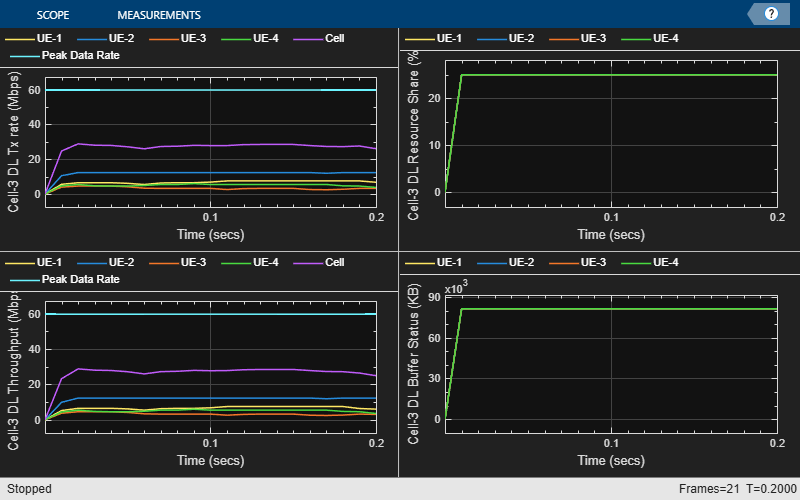Create objects to log MAC and PHY traces.

```linkDir = 0; % Indicates DL if enableTraces simSchedulingLogger = cell(simParameters.NumCells, 1); simPhyLogger = cell(simParameters.NumCells, 1); for cellIdx = 1:simParameters.NumCells simParameters.NCellID = simParameters.NCellIDList(cellIdx); % Create an object for MAC DL scheduling traces logging simSchedulingLogger{cellIdx} = hNRSchedulingLogger(simParameters, linkDir); % Create an object for PHY layer traces logging simPhyLogger{cellIdx} = hNRPhyLogger(simParameters); end end```

Store the index of cell ID of interest.

`cellOfInterestIdx = find(simParameters.CellOfInterest == simParameters.NCellIDList);`

Create an object for CQI and RB grid visualization

```if enableTraces && (simParameters.CQIVisualization || simParameters.RBVisualization) gridVisualizer = hNRGridVisualizer(simParameters, 'CellOfInterest', simParameters.CellOfInterest, ... 'MACLogger', simSchedulingLogger{cellOfInterestIdx}, 'VisualizationFlag', linkDir); end```

Create an object for MAC and PHY metrics visualization.

```nodes = struct('UEs', {UEs(cellOfInterestIdx, :)}, 'GNB', gNB{cellOfInterestIdx}); metricsVisualizer = hNRMetricsVisualizer(simParameters, 'CellOfInterest', simParameters.CellOfInterest, ... 'Nodes', nodes, 'EnableSchedulerMetricsPlots', true, 'EnablePhyMetricsPlots', true, 'VisualizationFlag', linkDir);```

Run processing loop

```slotNum = 0; numSymbolsSim = numSlotsSim * 14; % Simulation time in units of symbol duration (assuming normal cyclic prefix) % Execute all the symbols in the simulation for symbolNum = 1 : tickGranularity : numSymbolsSim if mod(symbolNum - 1, 14) == 0 slotNum = slotNum + 1; end % All the cells operating on same SCS hence slot durations are same for cellIdx = 1:simParameters.NumCells % For each cell % Run MAC and PHY layers of gNB run(gNB{cellIdx}); % Run MAC and PHY layers of UEs for ueIdx = 1:simParameters.NumUEs run(UEs{cellIdx, ueIdx}); end if enableTraces % MAC logging logCellSchedulingStats(simSchedulingLogger{cellIdx}, symbolNum, gNB{cellIdx}, UEs(cellIdx, :), linkDir); % PHY logging logCellPhyStats(simPhyLogger{cellIdx}, symbolNum, gNB{cellIdx}, UEs(cellIdx, :)); end end % Visualization % Check slot boundary if symbolNum > 1 && ((simParameters.SchedulingType == 1 && mod(symbolNum, 14) == 0) || (simParameters.SchedulingType == 0 && mod(symbolNum-1, 14) == 0)) % If the update periodicity is reached, plot scheduler metrics and PHY metrics at slot boundary if mod(slotNum, simParameters.MetricsStepSize) == 0 plotLiveMetrics(metricsVisualizer); end end for cellIdx = 1:simParameters.NumCells % Advance timer ticks for gNB and UEs by the number of symbols per slot advanceTimer(gNB{cellIdx}, tickGranularity); for ueIdx = 1:simParameters.NumUEs advanceTimer(UEs{cellIdx, ueIdx}, tickGranularity); end end end```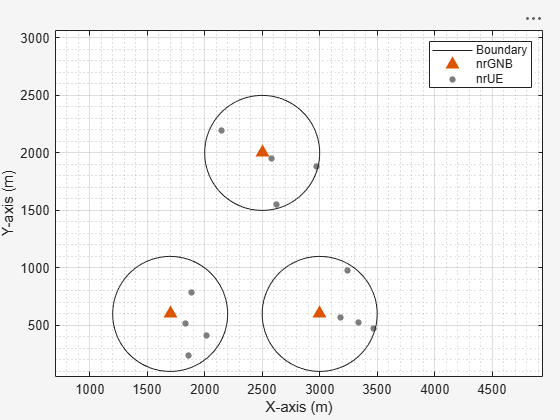Get the simulation metrics of the `simParameters.CellOfInterest` and save it in a MAT-file. The simulation metrics are saved in a MAT-file with the file name as `simulationMetricsFile`.

```metrics = getMetrics(metricsVisualizer); save(simulationMetricsFile, 'metrics');```

At the end of the simulation, the achieved value for system performance indicators is compared to their theoretical peak values (considering zero overheads). Performance indicators displayed are achieved data rate (UL and DL), achieved spectral efficiency (UL and DL), and BLER observed for UEs (DL and UL). The peak values are calculated as per 3GPP TR 37.910.

`displayPerformanceIndicators(metricsVisualizer);`
```Peak DL Throughput: 59.72 Mbps. Achieved Cell DL Throughput: 10.47 Mbps Achieved DL Throughput for each UE: [2.12 6.01 1.11 1.24] Achieved Cell DL Goodput: 10.33 Mbps Achieved DL Goodput for each UE: [2.07 6.01 1.05 1.2] Peak DL spectral efficiency: 5.97 bits/s/Hz. Achieved DL spectral efficiency for cell: 1.03 bits/s/Hz Block error rate for each UE in the downlink direction: [0.01 0 0.01 0.01] ```

### Simulation Visualization

The five types of run-time visualization shown are:

• Display of Network Topology: The figure shows the configured cell topology. For each cell, it shows the position of the gNB and the connected UEs.

• Display of CQI values for UEs over the PDSCH bandwidth: For details, see the 'Channel Quality Visualization' figure description in the NR PUSCH FDD Scheduling example.

• Display of resource grid assignment to UEs: The 2D time-frequency grid shows the resource allocation to the UEs. You can enable this visualization in the 'Logging and Visualization Configuration' section. For details, see the 'Resource Grid Allocation' figure description in the NR PUSCH FDD Scheduling example.

• Display of DL scheduling metrics plots: For details, see the 'Downlink Scheduler Performance Metrics ' figure description in the NR FDD Scheduling Performance Evaluation example.

• Display of DL Block Error Rates: For details, see the 'Block Error Rate (BLER) Visualization' figure description in the NR Cell Performance Evaluation with Physical Layer Integration example.

### Simulation Logs

The parameters used for simulation and the simulation logs are saved in MAT-files for post-simulation analysis and visualization. The simulation parameters are saved in a MAT-file with the file name as the value of configuration parameter `parametersLogFile`. The per time step logs, scheduling assignment logs, and BLER logs are captured for each cell in the simulation and saved in the MAT-file `simulationLogFile`. After the simulation, open the file to load `NCellID`, `DLTimeStepLogs`, `SchedulingAssignmentLogs`, and `BLERLogs` in the workspace.

NCellID: This stores the cell ID and represents cell to which the simulation logs belong.

DL Time step logs: Stores the per slot logs of the simulation with each slot as one row in the simulation. For details of log format, see the 'Simulation Logs' section of the NR PUSCH FDD Scheduling example.

Scheduling Assignment logs: Information of all the scheduling assignments and related information is logged in this file. For details of log format, see the 'Simulation Logs' section in the NR FDD Scheduling Performance Evaluation example.

BLER logs: Block error information observed in the simulation is logged in this file. For details of log format, see the 'BLER Logs' section in the NR Cell Performance Evaluation with Physical Layer Integration example.

You can run the script NRPostSimVisualization to get a post-simulation visualization of logs. For more details about the options to run this script, see the NR FDD Scheduling Performance Evaluation example.

```if enableTraces % Get the logs simulationLogs = cell(simParameters.NumCells, 1); for cellIdx = 1:simParameters.NumCells if(simParameters.DuplexMode == 0) % FDD logInfo = struct('NCellID', [], 'DLTimeStepLogs', [], 'SchedulingAssignmentLogs', [], 'BLERLogs', [], 'AvgBLERLogs', []); logInfo.DLTimeStepLogs = getSchedulingLogs(simSchedulingLogger{cellIdx}); else % TDD logInfo = struct('NCellID', [], 'TimeStepLogs', [], 'SchedulingAssignmentLogs', [], 'BLERLogs', [], 'AvgBLERLogs', []); logInfo.TimeStepLogs = getSchedulingLogs(simSchedulingLogger{cellIdx}); end logInfo.NCellID = simParameters.NCellIDList(cellIdx); logInfo.SchedulingAssignmentLogs = getGrantLogs(simSchedulingLogger{cellIdx}); % Scheduling assignments log logInfo.BLERLogs = getBLERLogs(simPhyLogger{cellIdx}); % BLER logs simulationLogs{cellIdx} = logInfo; end save(parametersLogFile, 'simParameters'); % Save simulation parameters in a MAT-file save(simulationLogFile, 'simulationLogs'); % Save simulation logs in a MAT-file end```

### Further Exploration

You can use this example to further explore these options:

• Model the uplink interference between the nodes by configuring uplink-related configuration. For details, see the NR Cell Performance Evaluation with Physical Layer Integration example.

• Model the Aggressor-Victim scenarios: The aggressor is the source of interference and the victim suffers due to the interference. Consider the DL scenario in the following figure. The macro cell UE is far away from the macro base station (BS) and near to the small cell. In DL direction, macro cell UE suffers from interference by the small cell BS. The small cell BS is called the aggressor and macro UE is called the victim.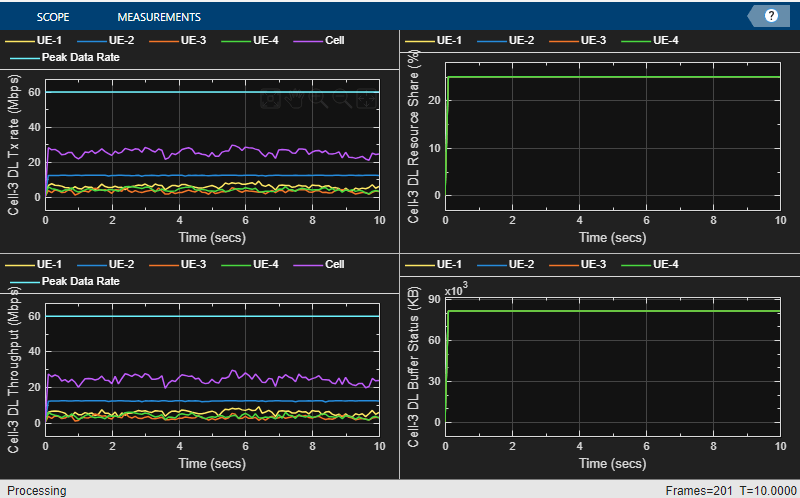• Model multiple clusters, where each cluster consists of cells operating on different frequencies, and analyze the impact of interference on the cell edge users.

• Model the effects of intra-cell mobility on the downlink metrics: The mobility model installed on the UE determines the pattern along which the UE moves within a particular cell. Set the `Mobility` field in the 'Derived Parameters' section to enable mobility for `ueOfInterest` in the `CellOfInterest`. The plot below demonstrates the effect of UE mobility on the goodput for a simulation duration of 1000 frames. The goodput decreases gradually as the UE moves away from the gNB. However, in comparison, the static UEs sustain their data rates throughout the simulation.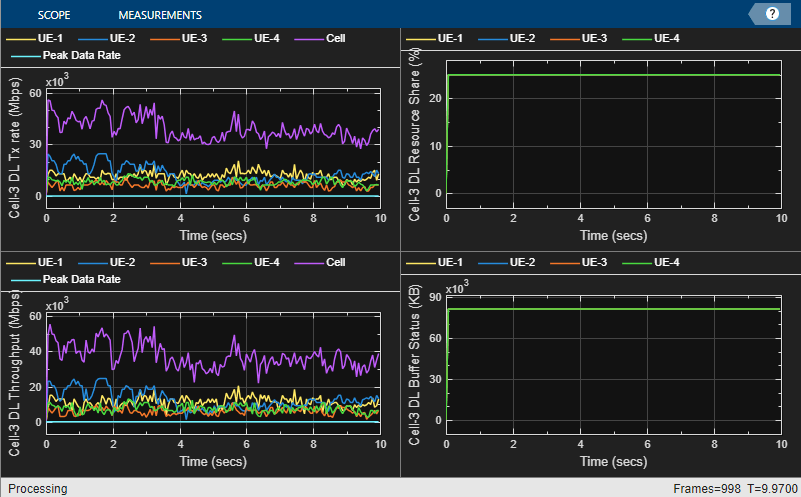Goodput plots for cell of interest with mobility installed on UE-2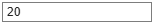Goodput plots for cell of interest with static UEs

Based on the described simulation parameters, the example evaluates the performance of the system measured in terms of various metrics. Different visualizations show the run time performance of the system. A more thorough post-simulation analysis by using the saved logs gives a detailed picture of the operations happening on a per slot basis.

### Local functions

```function plotNetwork(simParameters) % Create the figure figure('Name', 'Network Topology Visualization', 'units', 'normalized', 'outerposition', [0 0 1 1], 'Visible', "on"); title('Network Topology Visualization'); hold on; for cellIdx = 1:simParameters.NumCells % Plot the circle th = 0:pi/60:2*pi; xunit = simParameters.CellRadius * cos(th) + simParameters.GNBPosition(cellIdx, 1); yunit = simParameters.CellRadius * sin(th) + simParameters.GNBPosition(cellIdx, 2); if simParameters.CellOfInterest == simParameters.NCellIDList(cellIdx) h1 = plot(xunit, yunit, 'Color', 'green'); % Cell of interest else h2 = plot(xunit, yunit, 'Color', 'red'); end xlabel('X-Position (meters)') ylabel('Y-Position (meters)') % Add tool tip data for gNBs s1 = scatter(simParameters.GNBPosition(cellIdx, 1), simParameters.GNBPosition(cellIdx, 2), '^','MarkerEdgeColor', 'magenta'); cellIdRow = dataTipTextRow('Cell - ',{num2str(simParameters.NCellIDList(cellIdx))}); s1.DataTipTemplate.DataTipRows(1) = cellIdRow; posRow = dataTipTextRow('Position[X, Y]: ',{['[' num2str(simParameters.GNBPosition(cellIdx, :)) ']']}); s1.DataTipTemplate.DataTipRows(2) = posRow; % Add tool tip data for UEs uePosition = simParameters.UEPosition{cellIdx}; for ueIdx = 1:size(uePosition, 1) s2 = scatter(uePosition(ueIdx, 1), uePosition(ueIdx, 2), '.','MarkerEdgeColor', 'blue'); ueIdRow = dataTipTextRow('UE - ',{num2str(ueIdx)}); s2.DataTipTemplate.DataTipRows(1) = ueIdRow; posRow = dataTipTextRow('Position[X, Y]: ',{['[' num2str(uePosition(ueIdx, :)) ']']}); s2.DataTipTemplate.DataTipRows(2) = posRow; end end % Create the legend if simParameters.NumCells > 1 legend([h1 h2 s1 s2], 'Cell of interest', 'Interfering cells', 'gNodeB', 'UE', 'Location', 'northeastoutside') else legend([h1 s1 s2], 'Cell of interest', 'gNodeB', 'UE', 'Location', 'northeastoutside') end axis auto hold off; daspect([1000,1000,1]); % Set data aspect ratio end function uePositions = generateUEPositions(simParameters) % Return the position of UEs in each cell uePositions = cell(simParameters.NumCells, 1); for cellIdx=1:simParameters.NumCells gnbXCo = simParameters.GNBPosition(cellIdx, 1); % gNB X-coordinate gnbYCo = simParameters.GNBPosition(cellIdx, 2); % gNB Y-coordinate gnbZCo = zeros(simParameters.NumUEs, 1); % gNB Z-coordinate theta = rand(simParameters.NumUEs, 1)*(2*pi); % Expression to calculate position of UEs with in the cell. By default, % it will place the UEs randomly with in the cell r = sqrt(rand(simParameters.NumUEs, 1))*simParameters.CellRadius; x = round(gnbXCo + r.*cos(theta)); y = round(gnbYCo + r.*sin(theta)); uePositions{cellIdx} = [x y gnbZCo]; end end```

 3GPP TS 38.104. “NR; Base Station (BS) radio transmission and reception.” 3rd Generation Partnership Project; Technical Specification Group Radio Access Network.

 3GPP TS 38.214. “NR; Physical layer procedures for data.” 3rd Generation Partnership Project; Technical Specification Group Radio Access Network.

 3GPP TS 38.321. “NR; Medium Access Control (MAC) protocol specification.” 3rd Generation Partnership Project; Technical Specification Group Radio Access Network.

 3GPP TS 38.322. “NR; Radio Link Control (RLC) protocol specification.” 3rd Generation Partnership Project; Technical Specification Group Radio Access Network.

 3GPP TS 38.323. “NR; Packet Data Convergence Protocol (PDCP) specification.” 3rd Generation Partnership Project; Technical Specification Group Radio Access Network.

 3GPP TS 38.331. “NR; Radio Resource Control (RRC) protocol specification.” 3rd Generation Partnership Project; Technical Specification Group Radio Access Network.

 3GPP TR 37.910. “Study on self evaluation towards IMT-2020 submission.” 3rd Generation Partnership Project; Technical Specification Group Radio Access Network.